# Introduction to the MachineShop Package

### Package Version 1.5.0

University of Iowa
brian-j-smith@uiowa.edu

# 1 Description

MachineShop is a meta-package for statistical and machine learning with a unified interface for model fitting, prediction, performance assessment, and presentation of results. Support is provided for predictive modeling of numerical, categorical, and censored time-to-event outcomes and for resample (bootstrap, cross-validation, and split training-test sets) estimation of model performance. This vignette introduces the package interface with a survival data analysis example, followed by supported methods of variable specification; applications to other response variable types; available performance metrics, resampling techniques, and graphical and tabular summaries; and modeling strategies.

# 2 Features

• Unified and concise interface for model fitting, prediction, and performance assessment.
• Current support for 51 established models from 26 R packages.
• Dynamic model parameters.
• Ensemble modeling with stacked regression and super learners.
• Modeling of response variables types: binary factors, multi-class nominal and ordinal factors, numeric vectors and matrices, and censored time-to-event survival.
• Model specification with traditional formulas, design matrices, and flexible pre-processing recipes.
• Resample estimation of predictive performance, including cross-validation, bootstrap resampling, and split training-test set validation.
• Parallel execution of resampling algorithms.
• Choices of performance metrics: accuracy, areas under ROC and precision recall curves, Brier score, coefficient of determination (R2), concordance index, cross entropy, F score, Gini coefficient, unweighted and weighted Cohen’s kappa, mean absolute error, mean squared error, mean squared log error, positive and negative predictive values, precision and recall, and sensitivity and specificity.
• Graphical and tabular performance summaries: calibration curves, confusion matrices, partial dependence plots, performance curves, lift curves, and variable importance.
• Model tuning over automatically generated grids of parameter values and randomly sampled grid points.
• Model selection and comparisons for any combination of models and model parameter values.
• User-definable models and performance metrics.

# 3 Getting Started

## 3.1 Installation

# Current release from CRAN
install.packages("MachineShop")

# Development version from GitHub
# install.packages("devtools")
devtools::install_github("brian-j-smith/MachineShop")

# Development version with vignettes
devtools::install_github("brian-j-smith/MachineShop", build_vignettes = TRUE)

## 3.2 Documentation

Once installed, the following R commands will load the package and display its help system documentation. Online documentation and examples are available at the MachineShop website.

library(MachineShop)

# Package help summary
?MachineShop

# Vignette
RShowDoc("Introduction", package = "MachineShop")

# 4 Melanoma Example

The package is illustrated in the following sections with an overall survival analysis example in which the response variable is a time to event outcome. Since survival outcomes are a combination of numerical (time to event) and categorical (event) variables, package features for both variable types are illustrated in the example. Outcomes other than survival, including nominal and ordinal factors as well as numeric vectors and matrices, are supported by MachineShop and will be discussed.

Survival analysis is performed with the Melanoma dataset from the MASS package (Andersen et al. 1993). The dataset provides survival time, in days, from disease treatment to (1) death from disease, (2) alive at end of study, or (3) death from other causes for 205 Denmark patients with malignant melanomas. Also provided are potential predictors of the survival outcomes. The analysis begins by loading required packages MachineShop, survival, and MASS as well as magrittr (Bache and Wickham 2014) for its pipe (%>%) operator to simplify some of the code syntax. For the analysis, a binary overall survival outcome is created by combining the two death categories (1 and 3) into one.

## Analysis libraries
library(MachineShop)
library(survival)
library(MASS)
library(magrittr)

## Malignant melanoma analysis dataset
surv_df <- within(Melanoma, status <- as.numeric(status != 2))

Descriptive summaries of the study variables are given below in Table 1, followed by a plot of estimated overall survival probabilities and 95% confidence intervals.

Table 1. Variable summaries for the Melanoma survival analysis example.
Characteristic Value
Number of subjects 205
time
Median (Range) 2005 (10, 5565)
status
1 = Dead 71 (34.63%)
0 = Alive 134 (65.37%)
sex
1 = Male 79 (38.54%)
0 = Female 126 (61.46%)
age
Median (Range) 54 (4, 95)
year
Median (Range) 1970 (1962, 1977)
thickness
Median (Range) 1.94 (0.10, 17.42)
ulcer
1 = Presence 90 (43.9%)
0 = Absence 115 (56.1%)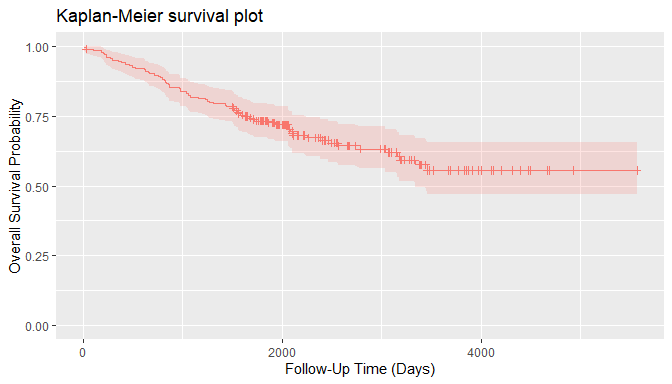For the analyses, the dataset is split into a training set on which a survival model will be fit and a test set on which predictions will be made and performance evaluated. A global formula surv_fo is defined to relate the predictors on the right hand side to the overall survival outcome on the left and will be used for all subsequent survival models.

## Training and test sets
set.seed(123)
train_indices <- sample(nrow(surv_df), nrow(surv_df) * 2 / 3)
surv_train <- surv_df[train_indices, ]
surv_test <- surv_df[-train_indices, ]

## Global formula for the analysis
surv_fo <- Surv(time, status) ~ sex + age + year + thickness + ulcer

# 5 Model Fit and Prediction

## 5.1 Model Information

Model fitting requires user specification of a MachineShop compatible model. A named list of package-supplied models can be obtained interactively with the modelinfo function, and includes the following components for each.

label
Character descriptor for the model.
packages
Character vector of source packages required to use the model. These need only be installed with the install.packages function or by equivalent means; but need not be loaded with, for example, the library function.
response_types
Character vector of response variable types supported by the model.
arguments
Closure with the argument names and corresponding default values of the model function.
grid
Logical indicating whether automatic generation of tuning parameter grids is implemented for the model.
varimp
Logical indicating whether variable importance is defined for the model.

Function modelinfo may be called without arguments, with one or more metric functions, an observed response variable, an observed and predicted response variable pair, response variable types, or resampled output; and will return information on all matching metrics.

## All available models
modelinfo() %>% names
#>   "AdaBagModel"         "AdaBoostModel"       "BARTModel"
#>   "BARTMachineModel"    "BlackBoostModel"     "C50Model"
#>   "CForestModel"        "CoxModel"            "CoxStepAICModel"
#>  "EarthModel"          "FDAModel"            "GAMBoostModel"
#>  "GBMModel"            "GLMBoostModel"       "GLMModel"
#>  "GLMStepAICModel"     "GLMNetModel"         "KNNModel"
#>  "LARSModel"           "LDAModel"            "LMModel"
#>  "MDAModel"            "NaiveBayesModel"     "NNetModel"
#>  "PDAModel"            "PLSModel"            "POLRModel"
#>  "QDAModel"            "RandomForestModel"   "RangerModel"
#>  "RPartModel"          "SelectedModel"       "StackedModel"
#>  "SuperModel"          "SurvRegModel"        "SurvRegStepAICModel"
#>  "SVMModel"            "SVMANOVAModel"       "SVMBesselModel"
#>  "SVMLaplaceModel"     "SVMLinearModel"      "SVMPolyModel"
#>  "SVMRadialModel"      "SVMSplineModel"      "SVMTanhModel"
#>  "TreeModel"           "TunedModel"          "XGBModel"
#>  "XGBDARTModel"        "XGBLinearModel"      "XGBTreeModel"

## Model-specific information
modelinfo(GBMModel)
#> $GBMModel #>$GBMModel$label #>  "Generalized Boosted Regression" #> #>$GBMModel$packages #>  "gbm" #> #>$GBMModel$response_types #>  "factor" "numeric" "Surv" #> #>$GBMModel$arguments #> function (distribution = NULL, n.trees = 100, interaction.depth = 1, #> n.minobsinnode = 10, shrinkage = 0.1, bag.fraction = 0.5) #> NULL #> #>$GBMModel$grid #>  TRUE #> #>$GBMModel$varimp #>  TRUE Information is displayed above for the GBMModel function — a generalized boosted regression model that can be applied to survival outcomes. ### 5.1.1 Type-Specific When data objects are supplied as arguments, information is returned on all models applicable to response variables of the same data types. If model functions are additionally supplied as arguments, information on the subset matching the data types is returned. ## All survival response-specific models modelinfo(Surv(0)) %>% names #>  "BARTModel" "BlackBoostModel" "CForestModel" #>  "CoxModel" "CoxStepAICModel" "GAMBoostModel" #>  "GBMModel" "GLMBoostModel" "GLMNetModel" #>  "RangerModel" "RPartModel" "SelectedModel" #>  "StackedModel" "SuperModel" "SurvRegModel" #>  "SurvRegStepAICModel" "TunedModel" ## Identify survival response-specific models modelinfo(Surv(0), CoxModel, GBMModel, SVMModel) %>% names #>  "CoxModel" "GBMModel" ### 5.1.2 Response Variable-Specific As a special case of type-specific arguments, existing response variables to be used in analyses may be given as arguments to identify applicable models. ## Models for a responses variable modelinfo(Surv(surv_df$time, surv_df$status)) %>% names #>  "BARTModel" "BlackBoostModel" "CForestModel" #>  "CoxModel" "CoxStepAICModel" "GAMBoostModel" #>  "GBMModel" "GLMBoostModel" "GLMNetModel" #>  "RangerModel" "RPartModel" "SelectedModel" #>  "StackedModel" "SuperModel" "SurvRegModel" #>  "SurvRegStepAICModel" "TunedModel" ## 5.2 Fit Function Package models, like GBMModel, can be specified in the model argument of the fit function to estimate a relationship (surv_fo) between predictors and an outcome based on a set of data (surv_train). Argument specifications may be in terms of the model function, function name, or a function call. ## Generalized boosted regression fit ## Model function surv_fit <- fit(surv_fo, data = surv_train, model = GBMModel) ## Model function name fit(surv_fo, data = surv_train, model = "GBMModel") ## Model function call fit(surv_fo, data = surv_train, model = GBMModel(n.trees = 100, interaction.depth = 1)) ## 5.3 Dynamic Model Parameters Dynamic model parameters are model function arguments defined as expressions to be evaluated at the time of model fitting. As such, their values can change based on the number of observations in the dataset supplied to the fit function. Expressions to dynamic parameters are specified within the package-supplied quote operator .() and can include the following objects: nobs number of observations in data. nvars number of predictor variables in data. y response variable. In the example below, Bayesian information criterion (BIC) based stepwise variable selection is performed by creating a CoxStepAICModel with dynamic parameter k to be calculated as the log number of observations in the fitted dataset. ## Dynamic model parameter k = log number of observations ## Number of observations: nobs fit(surv_fo, data = surv_train, model = CoxStepAICModel(k = .(log(nobs)))) ## Response variable: y fit (surv_fo, data = surv_train, model = CoxStepAICModel(k = .(log(length(y))))) ## 5.4 Predict Function A predict function is supplied for application to model fit results to obtain predicted values on a dataset specified with its newdata argument or on the original dataset if not specified. Survival means are predicted for survival outcomes by default. Estimates of the associated survival distributions are needed to calculate the means. For models, like GBMModel, that perform semi- or non-parametric survival analysis, Weibull approximations to the survival distributions are the default for mean estimation. Other choices of distributional approximations are exponential, Rayleigh, and empirical. Empirical distributions are applicable to Cox proportional hazards-based models and can be calculated with the method of Breslow (1972), Efron (1977, default), or Fleming and Harrington (1984). Note, however, that empirical survival means are undefined mathematically if an event does not occur at the longest follow-up time. In such situations, a restricted survival mean is calculated by changing the longest follow-up time to an event, as suggested by Efron (1967), which will be negatively biased. ## Predicted survival means (default: Weibull distribution) predict(surv_fit, newdata = surv_test) %>% head #>  776.3621 6568.9623 16476.4377 1301.2555 1650.2195 8737.8829 ## Predicted survival means (empirical distribution) predict(surv_fit, newdata = surv_test, dist = "empirical") %>% head #>  1059.962 4694.756 5273.094 1865.118 2351.706 4939.165 In addition to survival means, predicted survival probabilities (type = "prob") or 0-1 survival events (default: type = "response") can be obtained with the follow-up times argument. The cutoff probability for classification of survival events (or other binary responses) can be set optionally with the cutoff argument (default: cutoff = 0.5). As with mean estimation, distributional approximations to the survival functions may be specified for the predictions, with the default for survival probabilities being the empirical distribution. ## Predict survival probabilities and events at specified follow-up times surv_times <- 365 * c(5, 10) predict(surv_fit, newdata = surv_test, times = surv_times, type = "prob") %>% head #> Object of class "SurvProbs" #> Time 1 Time 2 #> [1,] 0.1211066 0.02533558 #> [2,] 0.8684308 0.78222860 #> [3,] 0.9569571 0.92625908 #> [4,] 0.3337804 0.14801706 #> [5,] 0.4439461 0.24320762 #> [6,] 0.9064022 0.84273837 #> Attribute "times": #>  1825 3650 predict(surv_fit, newdata = surv_test, times = surv_times, cutoff = 0.5) %>% head #> Object of class "SurvEvents" #> Time 1 Time 2 #> [1,] 1 1 #> [2,] 0 0 #> [3,] 0 0 #> [4,] 1 1 #> [5,] 1 1 #> [6,] 0 0 #> Attribute "times": #>  1825 3650 # 6 Variable Specifications Variable specification defines the relationship between response and predictor variables as well as the data used to estimate the relationship. Four main types of specifications are supported by the fit, resample, and tune functions: traditional formula, design matrix, model frame, and recipe. ## 6.1 Traditional Formula Variables may be specified with a traditional formula and data frame pair, as was done at the start of the survival example. This specification allows for allows for crossing (*), interaction (:), and removal (-) of predictors in the formula; . substitution of variables not already appearing in the formula; in-line functions of response variables; and in-lining of base R operators and math functions of predictors. ## Dataset library library(MASS) ## Formula specification fit(medv ~ ., data = Boston, model = GBMModel) ## 6.2 Design Matrix Variables stored separately in a design matrix of predictors and object of responses can be supplied to the fit functions directly. Fitting with design matrices has less computational overhead than traditional formulas and allows for greater numbers of predictor variables in some models, including GBMModel, GLMNetModel, and RandomForestModel. ## Example design matrix and response object x <- model.matrix(medv ~ . - 1, data = Boston) y <- Boston$medv

## Design matrix specification
fit(x, y, model = GBMModel)

## 6.3 Model Frame

A ModelFrame class is provided by the package for specification of predictor and response variables along with other attributes to control model fitting. Model frames can be specified in calls to the ModelFrame constructor function with a syntax similar to the traditional formula or design matrix.

## Model frame specification

## Formula
mf <- ModelFrame(medv ~ ., data = Boston)

fit(mf, model = GBMModel)

## Design matrix
mf <- ModelFrame(x, y)

fit(mf, model = GBMModel)

The model frame approach has a few advantages over model fitting directly with a traditional formula. One is that cases with missing values on any of the response or predictor variables are excluded from the model frame by default. This is often desirable for models that do not handle missing values. Conversely, missing values can be retained in the model frame by setting its argument na.rm = FALSE for models, like GBMModel, that do handle them. A second advantage is that case weights can be included in the model frame to be passed on to the model fitting functions.

## Model frame specification with case weights
mf <- ModelFrame(ncases / (ncases + ncontrols) ~ agegp + tobgp + alcgp, data = esoph,
weights = with(esoph, ncases + ncontrols))

fit(mf, model = GBMModel)

A third, which will be illustrated later, is user-specification of a variable for stratified resampling via the constructor’s strata argument.

## 6.4 Preprocessing Recipe

The recipes package (Kuhn and Wickham 2018) provides a flexible framework for defining predictor and response variables as well as preprocessing steps to be applied to them prior to model fitting. Using recipes helps ensure that estimation of predictive performance accounts for all modeling step. They are also a convenient way of consistently applying preprocessing to new data. A basic recipe is given below in terms of the formula and data frame ingredients needed for the analysis.

## Recipe specification
library(recipes)

rec <- recipe(medv ~ ., data = Boston)

fit(rec, model = GBMModel)

Case weights and stratified resampling are also supported for recipes via the designations of "case_weight" and "case_strata" roles, respectively. As an example, an initial step is included in the recipe below to update (alter) the role of variable weights to be case weights. That is followed by a step to convert three ordinal factors to integer scores.

## Recipe specification with case weights
df <- within(esoph, {
y <- ncases / (ncases + ncontrols)
weights <- ncases + ncontrols
})

rec <- recipe(y ~ agegp + tobgp + alcgp + weights, data = df) %>%
update_role(weights, new_role = "case_weight") %>%
step_ordinalscore(agegp, tobgp, alcgp)

fit(rec, model = GBMModel)

## 6.5 Summary

The variable specification approaches differ with respect to support for preprocessing, in-line functions, case weights, resampling strata, and computational overhead, as summarized in the table below. Only recipes apply preprocessing steps automatically during model fitting and should be used when it is important to account for such steps in the estimation of model predictive performance. Preprocessing would have to be done manually and separately otherwise. Design matrices have the lowest computational overhead and can enable analyses involving larger numbers of predictors than the other approaches. Both recipes and model frames allow for user-defined case weights (default: equal) and resampling strata (default: none). The remaining approaches default to equal weights and to strata defined by the response variable. Syntax ranges from simplest to most complex for design matrices, traditional formulas, model frames, and recipes, respectively. The relative strengths of each approach should be considered within the context of a given analysis when deciding upon which one to use.

Table 2. Characteristics of available variable specification approaches.
Specification Preprocessing In-line Functions Case Weights Resampling Strata Computational Overhead
Traditional Formula manual yes equal response medium
Design Matrix manual no equal response low
Model Frame
Traditional Formula manual yes user user medium
Design Matrix manual no user user low
Recipe automatic no user user high

# 7 Response Variable Types

The R class types of response variables play a central role in their analysis with the package. They determine, for example, the specific models that can be fit, fitting algorithms employed, predicted values produced, and applicable performance metrics and analyses. As described below, factors, ordered factors, numeric vectors and matrices, and survival responses are supported by the package.

## 7.1 Factors

Categorical responses with two or more levels should be coded as factor variables for analysis.

## Iris flowers species (3-level factor)
fit(Species ~ ., data = iris, model = GBMModel)
## Pima Indians diabetes statuses (binary factor)
library(MASS)

fit(type ~ ., data = Pima.tr, model = GBMModel)

## 7.2 Ordered Factors

Categorical responses can be designated as having ordered levels by storing them as ordered factor variables. For categorical vectors, this can be accomplished with the factor function and its argument ordered = TRUE or more simply with the ordered function. Numeric vectors can be converted to ordered factors with the cut function.

## Boston housing prices (ordered factor)
library(MASS)

df <- within(Boston, {
medv <- cut(medv, breaks = 3, ordered_result = TRUE)
})

fit(medv ~ ., data = df, model = GBMModel)

## 7.3 Numeric Vectors

Code univariate numerical responses as numeric variables.

## Boston housing prices
library(MASS)

fit(medv ~ ., data = Boston, model = GBMModel)

## 7.4 Numeric Matrices

Store multivariate numerical responses as numeric matrix variables for model fitting with traditional formulas or model frames.

## Anscombe's multiple regression models dataset

## Numeric matrix response formula
fit(cbind(y1, y2, y3) ~ x1, data = anscombe, model = LMModel)

For recipes, include the multiple response variables on the left hand side of the formula specification.

## Numeric matrix response recipe
rec <- recipe(y1 + y2 + y3 ~ x1, data = anscombe)

fit(rec, model = LMModel)

## 7.5 Survival Objects

Censored time-to-event survival responses should be stored as Surv variables for model fitting with traditional formulas or model frames.

## Survival response formula
library(survival)

fit(Surv(time, status) ~ ., data = surv_train, model = GBMModel)

For recipes, survival responses should be specified with the individual survival time and event variables given on the left hand side of the formula and with their roles designated as "surv_time" and "surv_event".

## Survival response recipe
rec <- recipe(time + status ~ ., data = surv_train) %>%
add_role(time, new_role = "surv_time") %>%
add_role(status, new_role = "surv_event")

fit(rec, model = GBMModel)

# 8 Model Performance Metrics

Performance metrics quantify associations between observed and predicted responses and provide a means of assessing and comparing the predictive performances of models.

## 8.1 Performance Function

Metrics can be computed with the performance function applied to observed responses and responses predicted with the predict function. In the case of observed versus predicted survival probabilities or events, metrics will be calculated at each survival time and returned along with their time-integrated mean.

## Survival performance metrics

## Observed responses
obs <- response(surv_fit, newdata = surv_test)

## Predicted survival means
pred_means <- predict(surv_fit, newdata = surv_test)
performance(obs, pred_means)
#>    CIndex
#> 0.6127013

## Predicted survival probabilities
pred_probs <- predict(surv_fit, newdata = surv_test, times = surv_times, type = "prob")
performance(obs, pred_probs)
#>     Brier.mean    Brier.time1    Brier.time2    ROCAUC.mean   ROCAUC.time1
#>      0.2700376      0.2265776      0.3134975      0.5350288      0.5965768
#>   ROCAUC.time2  Accuracy.mean Accuracy.time1 Accuracy.time2
#>      0.4734807      0.6548227      0.7349709      0.5746745

## Predicted survival events
pred_events <- predict(surv_fit, newdata = surv_test, times = surv_times)
performance(obs, pred_events)
#>  Accuracy.mean Accuracy.time1 Accuracy.time2
#>      0.6548227      0.7349709      0.5746745

Function performance computes a default set of metrics according to the observed and predicted response types, as indicated and in the order given in the table below.

Table 3. Default performance metrics by response types.

Response Default Metrics
Factor Brier Score, Accuracy, Cohen’s Kappa
Binary Factor Brier Score, Accuracy, Cohen’s Kappa, Area Under ROC Curve, Sensitivity, Specificity
Numeric Vector or Matrix Root Mean Squared Error, R2, Mean Absolute Error
Survival Means Concordance Index
Survival Probabilities Area Under ROC Curve, Brier Score, Accuracy
Survival Events Accuracy

These defaults may be changed by specifying one or more package-supplied metric functions to the metrics argument of performance. Specification of the metrics argument can be in terms of a single metric function, function name, or list of metric functions. List names, if specified, will be displayed as metric labels in graphical and tabular summaries; otherwise, the function names will be used as labels for unnamed lists.

## Single metric function
performance(obs, pred_means, metrics = cindex)

## Single metric function name
performance(obs, pred_means, metrics = "cindex")

## List of metric functions
performance(obs, pred_means, metrics = c(cindex, rmse, rmsle))

## Named list of metric functions
performance(obs, pred_means, metrics = c("CIndex" = cindex,
"RMSE" = rmse,
"RMSLE" = rmsle))

Metrics based on classification of two-level class probabilities, like sensitivity and specificity, optionally allow for specification of the classification cutoff probability (default: cutoff = 0.5).

## User-specified survival probability metrics
performance(obs, pred_probs, metrics = c(sensitivity, specificity), cutoff = 0.5)
#>  sensitivity.mean sensitivity.time1 sensitivity.time2  specificity.mean
#>         0.3569380         0.3470814         0.3667946         0.9323187
#> specificity.time1 specificity.time2
#>         0.8646375         1.0000000

## 8.2 Metric Functions

Whereas multiple package-supplied metrics can be calculated simultaneously with the performance function, each exists as a stand-alone function that can be called individually.

## Metric functions for survival means
cindex(obs, pred_means)
#>  0.6127013

rmse(obs, pred_means)
#>  5264.37

rmsle(obs, pred_means)
#>  1.934614

## Metric functions for survival probabilities
sensitivity(obs, pred_probs)
#>      mean     time1     time2
#> 0.3569380 0.3470814 0.3667946

specificity(obs, pred_probs)
#>      mean     time1     time2
#> 0.9323187 0.8646375 1.0000000

## 8.3 Metric Information

A named list of available metrics can be obtained interactively with the metricinfo function, and includes the following components for each one.

label
Character descriptor for the metric.
maximize
Logical indicating whether higher values of the metric correspond to better predictive performance.
arguments
Closure with the argument names and corresponding default values of the metric function.
response_types
Data frame of the observed and predicted response variable types supported by the metric.

Function metricinfo may be called without arguments, with one or more metric functions, an observed response variable, an observed and predicted response variable pair, response variable types, or resampled output; and will return information on all matching metrics.

## All available metrics
metricinfo() %>% names
#>   "accuracy"        "auc"             "brier"
#>   "cindex"          "cross_entropy"   "f_score"
#>   "fnr"             "fpr"             "gini"
#>  "kappa2"          "mae"             "mse"
#>  "msle"            "npv"             "ppv"
#>  "pr_auc"          "precision"       "r2"
#>  "recall"          "rmse"            "rmsle"
#>  "roc_auc"         "roc_index"       "rpp"
#>  "sensitivity"     "specificity"     "tnr"
#>  "tpr"             "weighted_kappa2"

## Metric-specific information
metricinfo(cindex)
#> $cindex #>$cindex$label #>  "Concordance Index" #> #>$cindex$maximize #>  TRUE #> #>$cindex$arguments #> function (observed, predicted = NULL, ...) #> NULL #> #>$cindex$response_types #> observed predicted #> 1 Resamples NULL #> 2 Surv numeric #> 3 factor numeric ### 8.3.1 Type-Specific When data objects are supplied as arguments, information is returned on all metrics applicable to response variables of the same data types. Observed response variable type is inferred from the first data argument and predicted type from the second, if given. For survival responses, predicted types may be numeric for survival means, SurvEvents for 0-1 survival events at specified follow-up times, or SurvProbs for follow-up time survival probabilities. If model functions are additionally supplied as arguments, information on the subset matching the data types is returned. ## Metrics for observed and predicted response variable types metricinfo(Surv(0)) %>% names #>  "accuracy" "auc" "brier" "cindex" "f_score" #>  "fnr" "fpr" "gini" "kappa2" "mae" #>  "mse" "msle" "npv" "ppv" "pr_auc" #>  "precision" "r2" "recall" "rmse" "rmsle" #>  "roc_auc" "roc_index" "rpp" "sensitivity" "specificity" #>  "tnr" "tpr" metricinfo(Surv(0), numeric(0)) %>% names #>  "cindex" "gini" "mae" "mse" "msle" "r2" "rmse" "rmsle" metricinfo(Surv(0), SurvEvents(0)) %>% names #>  "accuracy" "f_score" "fnr" "fpr" "kappa2" #>  "npv" "ppv" "precision" "recall" "roc_index" #>  "rpp" "sensitivity" "specificity" "tnr" "tpr" metricinfo(Surv(0), SurvProbs(0)) %>% names #>  "accuracy" "auc" "brier" "f_score" "fnr" #>  "fpr" "kappa2" "npv" "ppv" "pr_auc" #>  "precision" "recall" "roc_auc" "roc_index" "rpp" #>  "sensitivity" "specificity" "tnr" "tpr" ## Identify survival-specific metrics metricinfo(Surv(0), auc, cross_entropy, cindex) %>% names #>  "auc" "cindex" ### 8.3.2 Response Variable-Specific Existing response variables observed and those obtained from the predict function may be given as arguments to identify metrics that are applicable to them. ## Metrics for observed and predicted responses from model fits metricinfo(obs, pred_means) %>% names #>  "cindex" "gini" "mae" "mse" "msle" "r2" "rmse" "rmsle" metricinfo(obs, pred_probs) %>% names #>  "accuracy" "auc" "brier" "f_score" "fnr" #>  "fpr" "kappa2" "npv" "ppv" "pr_auc" #>  "precision" "recall" "roc_auc" "roc_index" "rpp" #>  "sensitivity" "specificity" "tnr" "tpr" ## 8.4 Factors Metrics applicable to multi-level factor response variables are summarized below. accuracy Proportion of correctly classified responses. brier Brier score. cross_entropy Cross entropy loss averaged over the number of cases. kappa2 Cohen’s kappa statistic measuring relative agreement between observed and predicted classifications. weighted_kappa2 Weighted Cohen’s kappa. This metric is only available for ordered factor responses. Brier score and cross entropy loss are computed directly on predicted class probabilities. The other metrics are computed on predicted class membership, defined as the factor level with the highest predicted probability. ## 8.5 Binary Factors Metrics for binary factors include those given for multi-level factors as well as the following. auc Area under a performance curve. cindex Concordance index computed as rank order agreement between predicted probabilities for paired event and non-event cases. This metric can be interpreted as the probability that a randomly selected event case will have a higher predicted value than a randomly selected non-event case, and is the same as area under the ROC curve. f_score F score, $$F_\beta = (1 + \beta^2) \frac{\text{precision} \times \text{recall}}{\beta^2 \times \text{precision} + \text{recall}}$$. F1 score $$(\beta = 1)$$ is the package default. fnr False negative rate, $$FNR = \frac{FN}{TP + FN} = 1 - TPR$$. Table 4. Confusion matrix of observed and predicted response classifications. Predicted Response Observed Response Negative Positive Negative True Negative (TN) False Negative (FN) Positive False Positive (FP) True Positive (TP) fpr False positive rate, $$FPR = \frac{FP}{TN + FP} = 1 - TNR$$. npv Negative predictive value, $$NPV = \frac{TN}{TN + FN}$$. ppv, precision Positive predictive value, $$PPV = \frac{TP}{TP + FP}$$. pr_auc, auc Area under a precision recall curve. roc_auc, auc Area under an ROC curve. roc_index A tradeoff function of sensitivity and specificity as defined by the f argument in this function (default: sensitivity + specificity). The function allows for specification of tradeoffs (Perkins and Schisterman 2006) other than the default of Youden’s J statistic (Youden 1950). rpp Rate of positive prediction, $$RPP = \frac{TP + FP}{TP + FP + TN + FN}$$. sensitivity, recall, tpr True positive rate, $$TPR =\frac{TP}{TP + FN} = 1 - FNR$$. specificity, tnr True negative rate, $$TNR = \frac{TN}{TN + FP} = 1 - FPR$$. Area under the ROC and precision-recall curves as well as the concordance index are computed directly on predicted class probabilities. The other metrics are computed on predicted class membership. Memberships are defined to be in the second factor level if predicted probabilities are greater than the function default or user-specified cutoff value. ## 8.6 Numerics Performance metrics are defined below for numeric vector responses. If applied to a numeric matrix response, the metrics are computed separately for each column and then averaged to produce a single value. gini Gini coefficient. mae Mean absolute error, $$MAE = \frac{1}{n}\sum_{i=1}^n|y_i - \hat{y}_i|$$, where $$y_i$$ and $$\hat{y}_i$$ are the $$n$$ observed and predicted responses. mse Mean squared error, $$MSE = \frac{1}{n}\sum_{i=1}^n(y_i - \hat{y}_i)^2$$. msle Mean squared log error, $$MSLE = \frac{1}{n}\sum_{i=1}^n(log(1 + y_i) - log(1 + \hat{y}_i))^2$$. r2 One minus residual divided by total sums of squares, $$R^2 = 1 - \sum_{i=1}^n(y_i - \hat{y}_i)^2 / \sum_{i=1}^n(y_i - \bar{y})^2$$. rmse Square root of mean squared error. rmsle Square root of mean squared log error. ## 8.7 Survival Objects All previously described metrics for binary factor responses—plus accuracy, Brier score and Cohen’s kappa—are applicable to survival probabilities predicted at specified follow-up times. Metrics are evaluated separately at each follow-up time and reported along with a time-integrated mean. The survival concordance index is computed with the method of Harrell (1982) and Brier score according to Graf et al. (1999); whereas, the others are computed according to the confusion matrix probabilities below, in which term $$\hat{S}(t)$$ is the predicted survival probability at follow-up time $$t$$ and $$T$$ is the survival time (Heagerty, Lumley, and Pepe 2004). Table 5. Confusion matrix of observed and predicted survival response classifications. Predicted Response Observed Response Non-Event Event Non-Event $$TN = \Pr(\hat{S}(t) \gt \text{cutoff} \cap T \ge t)$$ $$FN = \Pr(\hat{S}(t) \gt \text{cutoff} \cap T \lt t)$$ Event $$FP = \Pr(\hat{S}(t) \le \text{cutoff} \cap T \ge t)$$ $$TP = \Pr(\hat{S}(t) \le \text{cutoff} \cap T \lt t)$$ In addition, all of the metrics described for numeric vector responses are applicable to predicted survival means and are computed using only those cases with observed (non-censored) events. # 9 Resample Performance Estimation ## 9.1 Algorithms Model performance can be estimated with resampling methods that simulate repeated training and test set fits and predictions. With these methods, performance metrics are computed on each resample to produce an empirical distribution for inference. Resampling is controlled in the MachineShop with the functions: BootControl Simple bootstrap resampling (Efron and Tibshirani 1993). Models are repeatedly fit with bootstrap resampled training sets and used to predict the full data set. BootOptimismControl Optimism-corrected bootstrap resampling (Efron and Gong 1983; Harrell, Lee, and Mark 1996). CVControl Repeated K-fold cross-validation (Kohavi 1995). The full data set is repeatedly partitioned into K-folds. For a given partitioning, prediction is performed on each of the K folds with models fit on all remaining folds. OOBControl Out-of-bootstrap resampling. Models are fit with bootstrap resampled training sets and used to predict the unsampled cases. SplitControl Split training and test sets (Hastie, Tibshirani, and Friedman 2009). The data are randomly partitioned into a training and test set. TrainControl Training resubstitution. A model is fit on and used to predict the full training set in order to estimate training, or apparent, error (Efron 1986). For the survival example, repeated cross-validation control structures are defined to estimate model performance in predicting survival means and 5 and 10-year survival probabilities. In addition to arguments controlling the resampling algorithms, a seed can be set to ensure reproducibility of resampling results obtained with the structures. ## Control parameters for K-fold cross-validation ## Prediction of survival means surv_means_control <- CVControl(folds = 5, repeats = 3, seed = 123) ## Prediction of survival probabilities surv_probs_control <- CVControl(folds = 5, repeats = 3, times = surv_times, seed = 123) ## 9.2 Parallel Processing Resampling is implemented with the foreach package (Microsoft and Weston 2017) and will run in parallel if a compatible backend is loaded, such as that provided by the doParallel package (Microsoft and Weston 2018). ## Register multiple cores for parallel computations library(doParallel) registerDoParallel(cores = 2) ## 9.3 Resample Function Resampling is performed by calling the resample function with a variable specification, model, and control structure. Like the fit function, variables may be specified in terms of a traditional formula, design matrix, model frame, or recipe. ## Resample estimation for survival means and probabilities (res_means <- resample(surv_fo, data = surv_train, model = GBMModel, control = surv_means_control)) #> Object of class "Resamples" #> #> Models: GBMModel #> Stratification variable: (strata) #> #> Object of class "MLControl" #> #> Name: CVControl #> Label: K-Fold Cross-Validation #> Folds: 5 #> Repeats: 3 #> Seed: 123 (res_probs <- resample(surv_fo, data = surv_train, model = GBMModel, control = surv_probs_control)) #> Object of class "Resamples" #> #> Models: GBMModel #> Stratification variable: (strata) #> #> Object of class "MLControl" #> #> Name: CVControl #> Label: K-Fold Cross-Validation #> Folds: 5 #> Repeats: 3 #> Survival times: 1825, 3650 #> Seed: 123 ## 9.4 Summary Statistics The summary function when applied directly to output from resample computes summary statistics for the default performance metrics described in the Performance Function section. ## Summary of survival means metric summary(res_means) #> Mean Median SD Min Max NA #> CIndex 0.7610614 0.7663043 0.06966664 0.6256684 0.8924731 0 ## Summary of survival probability metrics summary(res_probs) #> Mean Median SD Min Max NA #> Brier.mean 0.1864575 0.1714480 0.05565866 0.12160556 0.3389423 0 #> Brier.time1 0.1686271 0.1674014 0.03595483 0.09871495 0.2322408 0 #> Brier.time2 0.2042879 0.1782289 0.08490057 0.11394700 0.4511018 0 #> ROCAUC.mean 0.7971056 0.7921197 0.06784720 0.65103433 0.9403871 0 #> ROCAUC.time1 0.8077798 0.8093603 0.07659626 0.68459040 0.9506220 0 #> ROCAUC.time2 0.7864314 0.7994861 0.06685039 0.61747827 0.9301522 0 #> Accuracy.mean 0.7162196 0.7030539 0.06184229 0.64424951 0.8497942 0 #> Accuracy.time1 0.7609229 0.7482143 0.05731050 0.66460905 0.8888889 0 #> Accuracy.time2 0.6715162 0.6652949 0.08388027 0.54285714 0.8477366 0 Other relevant metrics can be identified and summarized with the metricinfo and performance functions. ## Resample-specific metrics metricinfo(res_means) %>% names #>  "cindex" "gini" "mae" "mse" "msle" "r2" "rmse" "rmsle" ## User-specified survival means metrics summary(performance(res_means, metrics = c(cindex, rmse))) #> Mean Median SD Min Max NA #> cindex 0.7610614 0.7663043 6.966664e-02 0.6256684 0.8924731 0 #> rmse 4016.4176835 3642.1226845 1.586513e+03 1792.6746614 8511.1333117 0 Futhermore, summaries can be customized with a user-defined statistics function or list of statistics functions passed to the stats argument of summary. ## User-defined statistics function percentiles <- function(x) quantile(x, probs = c(0.25, 0.50, 0.75)) summary(res_means, stats = percentiles) #> 25% 50% 75% NA #> CIndex 0.720087 0.7663043 0.8113255 0 ## User-defined list of statistics functions summary(res_means, stats = c(Mean = mean, Percentile = percentiles)) #> Mean Percentile.25% Percentile.50% Percentile.75% NA #> CIndex 0.7610614 0.720087 0.7663043 0.8113255 0 ## 9.5 Plots Summary plots of resample output can be obtained with the plot function. Boxplots are the default plot type; but density, errorbar, and violin plots are also available. Plots are generated with the ggplot2 package (Wickham 2016) and returned as ggplot objects. As such, annotation and formatting defined for ggplots can be applied to the returned plots. ## Libraries for plot annotation and fomatting library(ggplot2) library(gridExtra) ## Individual ggplots p1 <- plot(res_means) p2 <- plot(res_means, type = "density") p3 <- plot(res_means, type = "errorbar") p4 <- plot(res_means, type = "violin") ## Grid of plots grid.arrange(p1, p2, p3, p4, nrow = 2)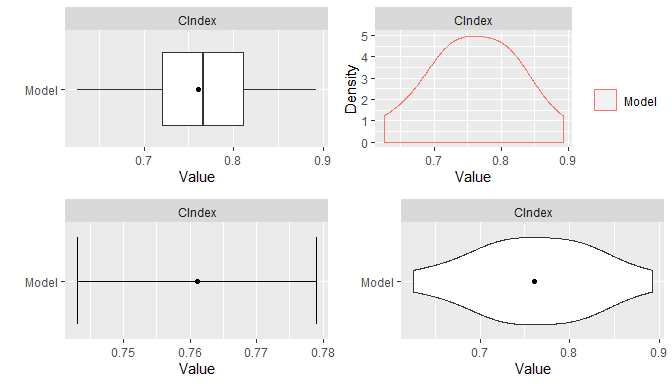## 9.6 Stratified Resampling Stratification of cases for the construction of resampled training and test sets can be employed to help achieve balance across the sets. Stratified resampling is automatically performed if variable specification is in terms of a traditional formula and will be done according to the response variable if a numeric vector or factor, the event variable if survival, and the first variable if a numeric matrix. For model frames and recipes, stratification variables must be defined explicitly with the strata argument to the ModelFrame constructor or with the "case_strata" role designation in a recipe step. ## Model frame with case status stratification mf <- ModelFrame(surv_fo, data = surv_train, strata = surv_train$status)

resample(mf, model = GBMModel)

## Recipe with case status stratification
rec <- recipe(time + status ~ ., data = surv_train) %>%
add_role(time, new_role = "surv_time") %>%
add_role(status, new_role = "surv_event") %>%
add_role(status, new_role = "case_strata")

resample(rec, model = GBMModel)

## 9.7 Dynamic Model Parameters

As discussed previously in the Model Fit and Prediction section, dynamic model parameters are evaluated at the time of model fitting and can depend on the number of observations in the fitted dataset. In the context of resampling, dynamic parameters are repeatedly evaluated at each fit of the resampled datasets. As such, their values can change based on the observations selected for training at each iteration of the resampling algorithm.

## Dynamic model parameter k = log number of training set observations
resample(surv_fo, data = surv_train, model = CoxStepAICModel(k = .(log(nobs))))

## 9.8 Model Comparisons

Resampled metrics from different models can be combined for comparison with the Resamples function. Optional names given on the left hand side of equal operators within calls to Resamples will be used as labels in output from the summary and plot functions. For comparisons of resampled output, the same control structure must be used in all associated calls to resample to ensure that resulting model metrics are computed on the same resampled training and test sets. The combined resample output can be summarized and plotted as usual.

## Resample estimation
res1 <- resample(surv_fo, data = surv_train, model = GBMModel(n.trees = 25),
control = surv_means_control)
res2 <- resample(surv_fo, data = surv_train, model = GBMModel(n.trees = 50),
control = surv_means_control)
res3 <- resample(surv_fo, data = surv_train, model = GBMModel(n.trees = 100),
control = surv_means_control)

## Combine resample output for comparison
(res <- Resamples(GBM1 = res1, GBM2 = res2, GBM3 = res3))
#> Object of class "Resamples"
#>
#> Models: GBM1, GBM2, GBM3
#> Stratification variable: (strata)
#>
#> Object of class "MLControl"
#>
#> Name: CVControl
#> Label: K-Fold Cross-Validation
#> Folds: 5
#> Repeats: 3
#> Seed: 123

summary(res)
#> , , CIndex
#>
#>           Mean    Median         SD       Min       Max NA
#> GBM1 0.7738602 0.7743590 0.08222939 0.6096257 0.9354839  0
#> GBM2 0.7730294 0.7692308 0.07206580 0.6363636 0.9354839  0
#> GBM3 0.7610614 0.7663043 0.06966664 0.6256684 0.8924731  0

plot(res)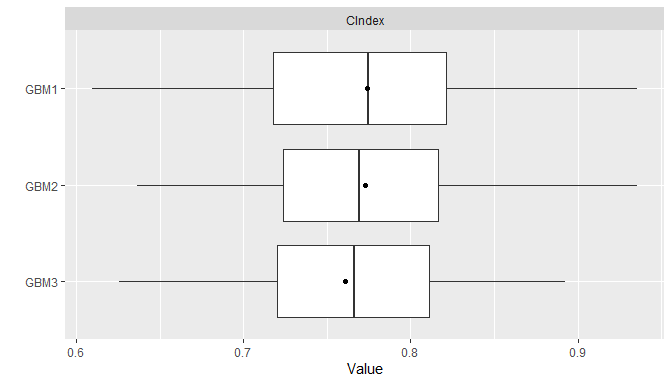Pairwise model differences for each metric can be calculated with the diff function applied to results from a call to Resamples. Resulting differences can be summarized descriptively with the summary and plot functions and assessed for statistical significance with the t.test function.

## Pairwise model comparisons
(perfdiff <- diff(res))
#> Object of class "PerformanceDiff"
#>
#> Metrics: CIndex
#> Models: GBM1 - GBM2, GBM1 - GBM3, GBM2 - GBM3

summary(perfdiff)
#> , , CIndex
#>
#>                     Mean      Median         SD         Min        Max NA
#> GBM1 - GBM2 0.0008308229 0.005128205 0.01863138 -0.03482587 0.02331606  0
#> GBM1 - GBM3 0.0127988563 0.008982036 0.02271720 -0.01990050 0.05434783  0
#> GBM2 - GBM3 0.0119680334 0.010695187 0.01992512 -0.02590674 0.04891304  0

plot(perfdiff)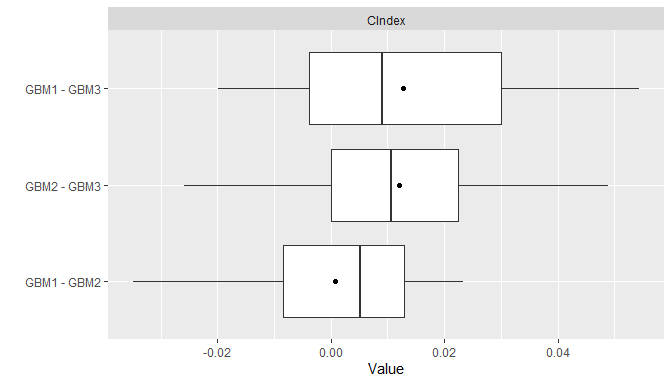t.test(perfdiff)
#> Object of class "PerformanceDiffTest"
#>
#> Upper diagonal: mean differences (row - column)
#> Lower diagonal: p-values
#> P-value adjustment method: holm
#>
#> , , CIndex
#>
#>           GBM1         GBM2       GBM3
#> GBM1        NA 0.0008308229 0.01279886
#> GBM2 0.8653540           NA 0.01196803
#> GBM3 0.1065908 0.1065908159         NA

# 10 Performance Analyses

Calculation of performance metrics on test sets or by resampling, as discussed previously, is one method of assessing model performance. Others available include measures of predictor variable importance, calibration curves comparing observed and predicted response values, partial dependence plots, and receiver operating characteristic analysis.

## 10.1 Variable Importance

The importance of variables in a model fit is estimated with the varimp function and plotted with plot. Variable importance is a relative measure of the contributions of model predictors and has a default range of 0 to 100, where 0 denotes the least important variables and 100 the most. Classes of models can differ with respect to how variable importance is defined. In the case of a GBMModel, importance of each predictor is based on the sum of squared empirical improvements over all internal tree nodes created by splitting on that variable (Greenwell et al. 2019).

## Predictor variable importance
(vi <- varimp(surv_fit))
#>             Overall
#> age       100.00000
#> thickness  96.67023
#> year       54.34984
#> ulcer      25.68188
#> sex         0.00000

plot(vi)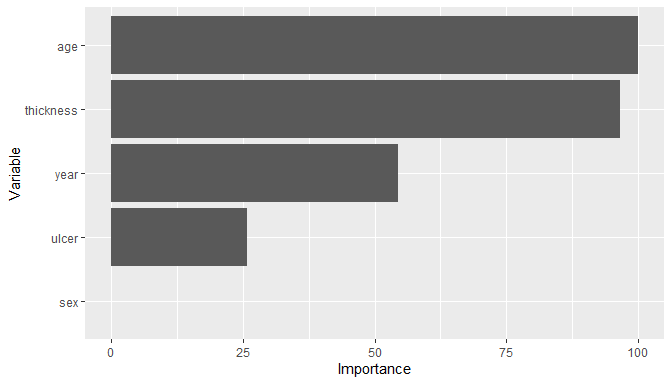Alternatively, importance is based on one minus predictor variable p-values for statistical models, like CoxModel, that produce them. For other classes of models, variable importance is generally defined and calculated by their underlying source packages.

## 10.2 Calibration Curves

Agreement between model-predicted and observed values can be visualized with calibration curves. In the construction of these curves, cases are partitioned into equally spaced bins according to their (resampled) predicted responses. Mean observed responses are then calculated within each of the bins and plotted on the vertical axis against the bin midpoints on the horizontal axis.

## Binned calibration curves
cal <- calibration(res_probs, breaks = 10)
plot(cal, se = TRUE)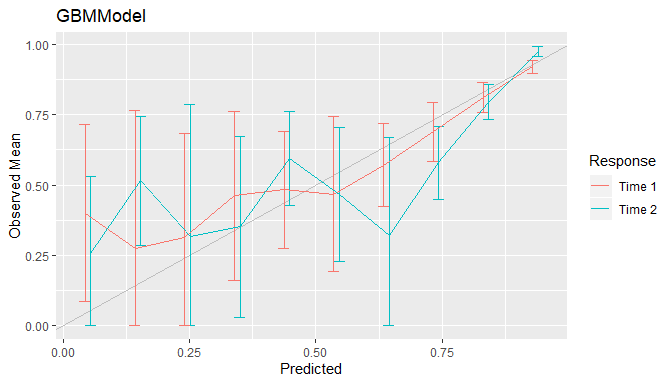As an alternative to discrete bins, curves can be smoothed over the individual predicted values by setting breaks = NULL.

## Smoothed calibration curves
cal <- calibration(res_probs, breaks = NULL)
plot(cal)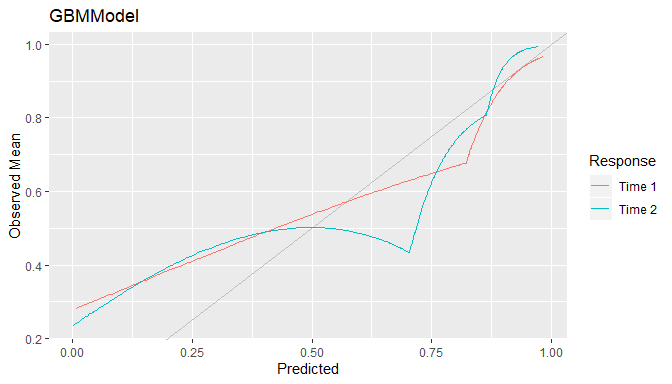Calibration curves that are close to the 45$$^\circ$$ line indicate close agreement between observed and predicted responses and a model that is said to be well calibrated.

## 10.3 Confusion Matrices

Confusion matrices of cross-classified observed and predicted categorical responses are available with the confusion function. They can be constructed with predicted class membership or with predicted class probabilities. In the latter case, predicted class membership is derived from predicted probabilities according to a probability cutoff value for binary factors (default: cutoff = 0.5) and according to the class with highest probability for factors with more than two levels.

## Confusion matrices
(conf <- confusion(res_probs, cutoff = 0.5))
#> GBMModel.time1 :
#>          Observed
#> Predicted         0         1
#>         0 262.94463  64.05537
#>         1  32.57143  48.42857
#>
#> GBMModel.time2 :
#>          Observed
#> Predicted         0         1
#>         0 201.49597  82.50403
#>         1  49.85001  74.14999
plot(conf)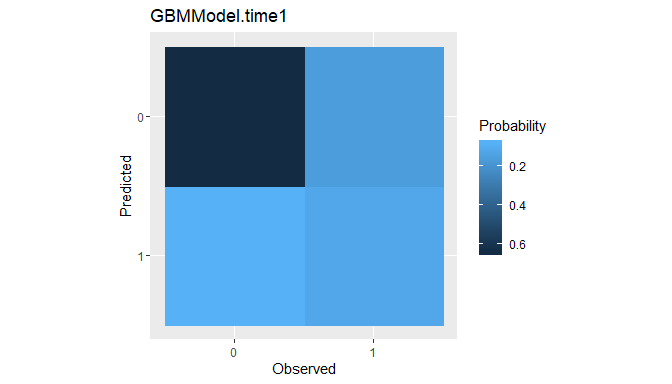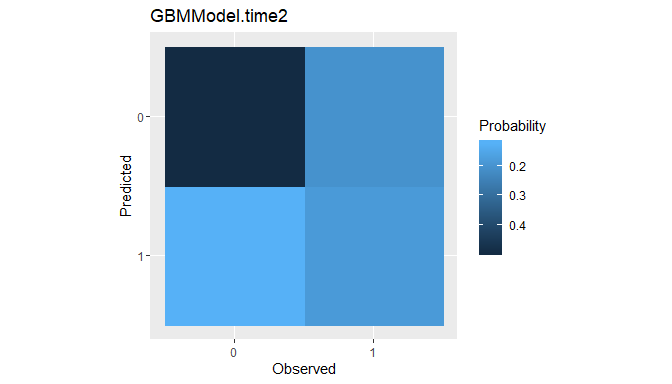Confusion matrices are the data structure upon which many of the performance metrics described earlier for factor predictor variables are based. Metrics commonly reported for confusion matrices are generated by the summary function.

## Summary performance metrics
summary(conf)
#> GBMModel.time1 :
#> Number of responses: 408
#> Accuracy (SE): 0.7631696 (0.02104743)
#> Majority class: 0.7243041
#> Kappa: 0.3507188
#>
#>                     0         1
#> Observed    0.7243041 0.2756959
#> Predicted   0.8014706 0.1985294
#> Agreement   0.6444721 0.1186975
#> Sensitivity 0.8897812 0.4305376
#> Specificity 0.4305376 0.8897812
#> PPV         0.8041120 0.5978836
#> NPV         0.5978836 0.8041120
#>
#> GBMModel.time2 :
#> Number of responses: 408
#> Accuracy (SE): 0.6756028 (0.02317684)
#> Majority class: 0.616044
#> Kappa: 0.2862432
#>
#>                     0         1
#> Observed    0.6160440 0.3839560
#> Predicted   0.6960784 0.3039216
#> Agreement   0.4938627 0.1817402
#> Sensitivity 0.8016678 0.4733360
#> Specificity 0.4733360 0.8016678
#> PPV         0.7094928 0.5979838
#> NPV         0.5979838 0.7094928

Summaries can also be obtained with performance function but for select metrics specified by the user.

## Confusion matrix-specific metrics
metricinfo(conf) %>% names
#>   "accuracy"    "f_score"     "fnr"         "fpr"         "kappa2"
#>   "npv"         "ppv"         "precision"   "recall"      "roc_index"
#>  "rpp"         "sensitivity" "specificity" "tnr"         "tpr"

## User-specified metrics
performance(conf, metrics = c("Accuracy" = accuracy,
"Sensitivity" = sensitivity,
"Specificity" = specificity))
#> GBMModel.time1 :
#>    Accuracy Sensitivity Specificity
#>   0.7631696   0.4305376   0.8897812
#>
#> GBMModel.time2 :
#>    Accuracy Sensitivity Specificity
#>   0.6756028   0.4733360   0.8016678

## 10.4 Partial Dependence Plots

Partial dependence plots display the marginal effects of predictors on a response variable. Dependence for a select set of one or more predictor variables $$X_S$$ is computed as $\bar{f}_S(X_S) = \frac{1}{N}\sum_{i=1}^N f(X_S, x_{iS'}),$ where $$f$$ is a fitted prediction function and $$x_{iS'}$$ are values of the remaining predictors in a dataset of $$N$$ cases. The response scale displayed in dependence plots will depend on the response variable type: probability for predicted factors and survival probabilities, original scale for numerics, and survival time for predicted survival means. By default, dependence is computed for each select predictor individually over a grid of 10 approximately evenly spaced values and averaged over the dataset on which the prediction function was fit.

## Partial dependence plots
pd <- dependence(surv_fit, select = c(thickness, age))
plot(pd)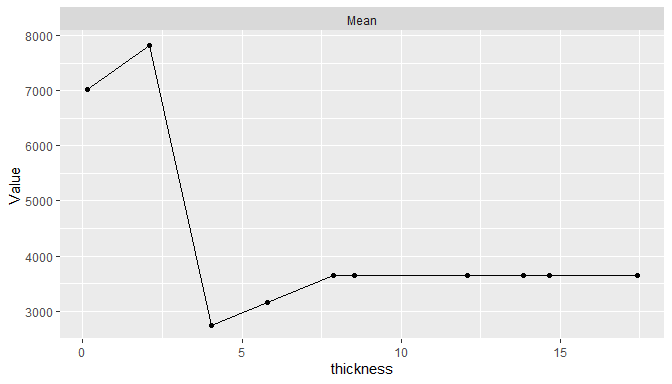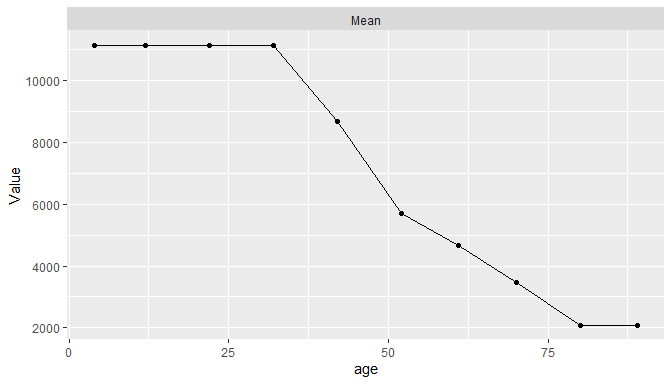Averaging may be performed over different datasets to estimate marginal effects in other populations of cases, over different numbers of predictor values, and over quantile spacing of the values.

pd <- dependence(surv_fit, data = surv_test, select = thickness, n = 20,
intervals = "quantile")
plot(pd)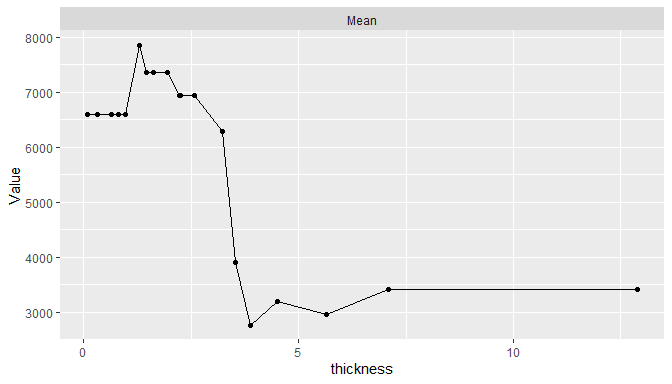In addition, dependence may be computed for combinations of multiple predictors to examine interaction effects and for summary statistics other than the mean.

## 10.5 Performance Curves

Tradeoffs between correct and incorrect classifications of binary outcomes, across the range of possible cutoff probabilities, can be studied with performance curves.

### 10.5.1 ROC

Receiver operating characteristic (ROC) curves are one example in which true positive rates (sensitivity) are plotted against false positive rates (1 - specificity) (Fawcett 2006). Higher ROC curves are indicative of better predictive performance.

## ROC curves
roc <- performance_curve(res_probs)
plot(roc, diagonal = TRUE)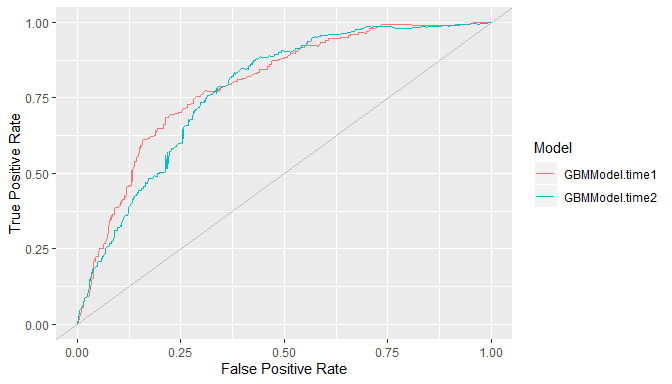ROC curves show the relation between the two rates being plotted but not their relationships with specific cutoff values. The latter may be helpful for the selection of a cutoff value to apply in practice. Accordingly, separate plots of each rate versus the range of possible cutoff values are available with the type = "cutoffs" option.

plot(roc, type = "cutoffs")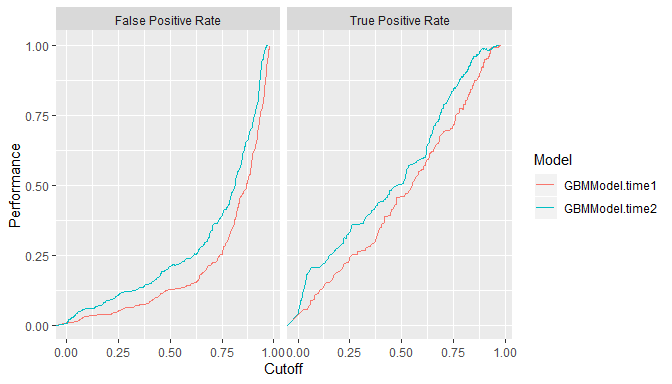Area under resulting ROC curves can be computed as an overall measure of model predictive performance and interpreted as the probability that a randomly selected event case will have a higher predicted value than a randomly selected non-event case.

auc(roc)
#> Model: GBMModel.time1
#>  0.7937907
#> --------------------------------------------------------
#> Model: GBMModel.time2
#>  0.7760905

### 10.5.2 Precision Recall

In general, any two binary response metrics may be specified for the construction of a performance curve. Precision recall curves are another example (Davis and Goadrich 2006).

## Precision recall curves
pr <- performance_curve(res_probs, metrics = c(precision, recall))
plot(pr)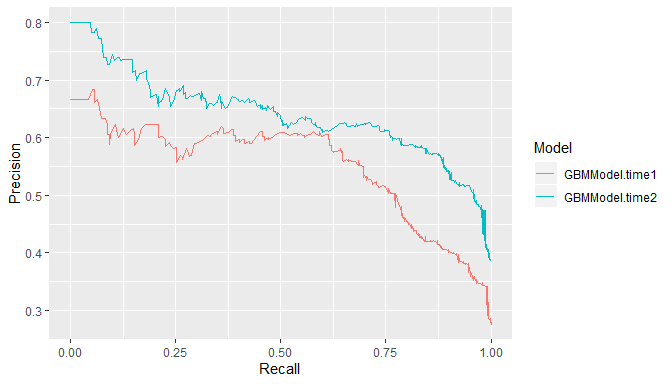auc(pr)
#> Model: GBMModel.time1
#>  0.5525249
#> --------------------------------------------------------
#> Model: GBMModel.time2
#>  0.6405763

### 10.5.3 Lift

Lift curves depict the rate at which observed binary responses are identifiable from (resampled) predicted response probabilities. In particular, they plot the true positive findings (sensitivity) against the positive test rates for all possible classification probability cutoffs. Accordingly, a lift curve can be interpreted as the rate at which positive responses are found as a function of the positive test rate among cases.

## Lift curves
lf <- lift(res_probs)
plot(lf, find = 0.75)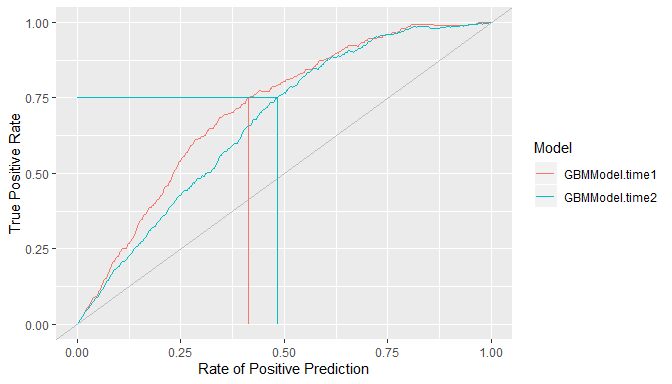# 11 Modeling Strategies

## 11.1 Model Tuning

Many of the modeling functions have arguments, or tuning parameters, that control aspects of their model fitting algorithms. For example, GBMModel parameters n.trees and interaction.depth control the number of decision trees to fit and the maximum depth of variable interactions. The tune function performs model fitting over a grid of parameter values and returns the model with the most optimal values. Optimality is determined based on the first performance metric of the metrics argument of tune if supplied or the first default metric of the performance function otherwise. Argument grid additionally controls the construction of grid values and can be a single numeric value giving the grid length in each parameter dimension. As shown in the output below, tuning results include the tuning parameter grid values, the names of models fit to each, all calculated metrics, the final model selected, the metric upon which its selection was based, and its tuning parameters.

## Tune over automatic grid of model parameters
(tuned_model <- tune(surv_fo, data = surv_train, model = GBMModel,
grid = 3,
control = surv_means_control,
metrics = c("CIndex" = cindex, "RMSE" = rmse)))
#> Object of class "MLModelTune"
#>
#> Model name: GBMModel
#> Label: Generalized Boosted Regression
#> Packages: gbm
#> Response types: factor, numeric, Surv
#>
#> Parameters:
#> $n.trees #>  50 #> #>$interaction.depth
#>  1
#>
#> $n.minobsinnode #>  10 #> #>$shrinkage
#>  0.1
#>
#> \$bag.fraction
#>  0.5
#>
#> Grid:
#>   n.trees interaction.depth
#> 1      50                 1
#> 2     100                 1
#> 3     150                 1
#> 4      50                 2
#> 5     100                 2
#> 6     150                 2
#> 7      50                 3
#> 8     100                 3
#> 9     150                 3
#>
#> Object of class "Performance"
#>
#> Metrics: CIndex, RMSE
#> Models: GBMModel.1, GBMModel.2, GBMModel.3, GBMModel.4, GBMModel.5, GBMModel.6, GBMModel.7, GBMModel.8, GBMModel.9
#>
#> Selected (CIndex): GBMModel.1

Grid values may also be a call to Grid with the grid length and number of grid points to sample at random or a user-specified data frame of grid points.

## Tune over randomly sampled grid points
tune(surv_fo, data = surv_train, model = GBMModel,
grid = Grid(length = 100, random = 10),
control = surv_means_control)

## Tune over user-specified grid points
tune(surv_fo, data = surv_train, model = GBMModel,
grid = expand.grid(n.trees = c(25, 50, 100),
interaction.depth = 1:3),
control = surv_means_control)

Statistics summarizing the resampled performance metrics across all tuning parameter combinations can be obtained with the summary function.

summary(tuned_model)
#> , , CIndex
#>
#>                 Mean    Median         SD       Min       Max NA
#> GBMModel.1 0.7730294 0.7692308 0.07206580 0.6363636 0.9354839  0
#> GBMModel.2 0.7610614 0.7663043 0.06966664 0.6256684 0.8924731  0
#> GBMModel.3 0.7564966 0.7647059 0.06743686 0.6480447 0.8870968  0
#> GBMModel.4 0.7687377 0.7616580 0.08218605 0.6256983 0.9301075  0
#> GBMModel.5 0.7629211 0.7616580 0.07106607 0.6592179 0.9032258  0
#> GBMModel.6 0.7562730 0.7409326 0.07500287 0.5865922 0.8602151  0
#> GBMModel.7 0.7608822 0.7487685 0.07981747 0.5989305 0.8978495  0
#> GBMModel.8 0.7514888 0.7700535 0.06886940 0.6201117 0.8494624  0
#> GBMModel.9 0.7443015 0.7564767 0.06410204 0.6312849 0.8324324  0
#>
#> , , RMSE
#>
#>                 Mean   Median       SD      Min       Max NA
#> GBMModel.1  3818.182 3835.515 1251.954 1992.999  5953.100  0
#> GBMModel.2  4016.418 3642.123 1586.513 1792.675  8511.133  0
#> GBMModel.3  4226.311 3790.948 1873.500 1342.394  8857.896  0
#> GBMModel.4  5614.160 4428.054 3009.006 1912.743 11666.415  0
#> GBMModel.5  5407.976 3893.101 3288.373 1419.643 14090.833  0
#> GBMModel.6  5450.541 5941.670 2733.285 1535.649 10480.901  0
#> GBMModel.7  7922.834 6177.645 4506.633 2941.312 16243.705  0
#> GBMModel.8 10728.876 7384.484 7889.461 2564.302 26325.938  0
#> GBMModel.9 11470.890 9691.195 7191.709 1999.900 26402.048  0

Line plots of tuning results display the resampled metric means, or another statistic specified with the stat argument, versus the first tuning parameter values and with lines grouped according to the remaining parameters, if any.

plot(tuned_model, type = "line")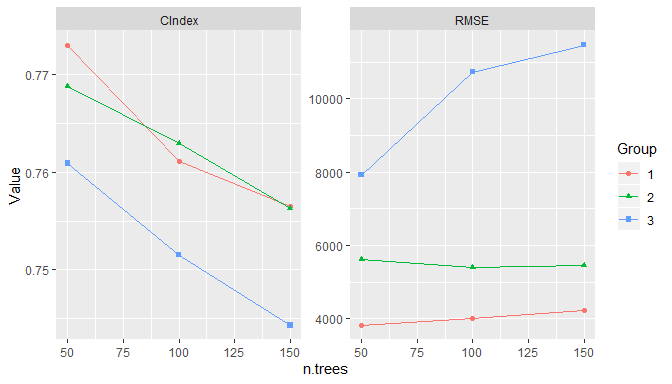The return value of tune is a model object with the optimal tuning parameters and not a model fit object. The returned model can be fit subsequently to a set of data with the fit function. Having the final model allows for fitting to the same dataset on which tuning was performed or to datasets from other case populations.

## Fit the tuned model
surv_fit <- fit(surv_fo, data = surv_train, model = tuned_model)

As illustrated in the examples above, the tune function allows for comparisons of all models fit to the parameter grid points and returns the optimal model for subsequent fitting. For applications in which fitting, performance assessment, and prediction with the optimal model are of primary interest, the TunedModel function is provided as a model interface to the tune function. The model interface has the advantage of enabling predictive performance estimation, with the resample function, that accounts for the full tuning grid process.

## Model interface for grid tuning
tuned_model <- TunedModel(GBMModel, grid = 3, control = surv_means_control,
metrics = c("CIndex" = cindex, "RMSE" = rmse))
surv_fit <- fit(surv_fo, data = surv_train, model = tuned_model)
(vi <- varimp(surv_fit))
#>             Overall
#> thickness 100.00000
#> age        83.50962
#> year       39.81938
#> ulcer      29.61630
#> sex         0.00000

## Predictive performance of the tuning process
res_tuned <- resample(surv_fo, data = surv_train, model = tuned_model,
control = surv_means_control)
summary(performance(res_tuned))
#>             Mean    Median         SD      Min       Max NA
#> CIndex 0.7692781 0.7604167 0.06627141 0.657754 0.8924731  0

## 11.2 Model Selection

Model selection can be conducted with the tune or SelectedModel function to automatically choose from any combination of models and model parameters. Selection has as a special case the just-discussed tuning of a single model over a grid of parameter values. Combinations of model functions, function names, or function calls can be supplied as lists to the models argument of tune or as arguments to SelectedModel in order to define sets of candidate models from which to select. An expand.model helper function is additionally available to expand a model over a grid of tuning parameters for inclusion in the candidate set if so desired. In this general form of model selection, the grid argument in the tuning functions is not used.

## Model interface for model selection
selected_model <- SelectedModel(
expand.model(GBMModel, n.trees = c(50, 100), interaction.depth = 1:2),
GLMNetModel(lambda = 0.01),
CoxModel,
SurvRegModel
)

## Fit the selected model
fit(surv_fo, data = surv_train, model = selected_model)

Selection may also be performed over candidate sets that include tuned models. For instance, the SelectedModel function is applicable to sets containing different classes of models each individually tuned over a grid of parameters.

## Model interface for selection among tuned models
selected_tuned_model <- SelectedModel(
TunedModel(GBMModel, control = surv_means_control),
TunedModel(GLMNetModel, control = surv_means_control),
TunedModel(CoxModel, control = surv_means_control)
)

## Fit the selected tuned model
fit(surv_fo, data = surv_train, model = selected_tuned_model)

## 11.3 Ensemble Models

Ensemble modelling methods combine $$m = 1, \ldots, M$$ base learning models as a strategy to improve predictive performance. Two methods implemented in Machineshop are stacked regression (Breiman 1996) and super learners (van der Lann and Hubbard 2007). Stacked regression fits a linear combination of predictions from specified base learners to produce a prediction function of the form $\hat{f}(x) = \sum_{m=1}^M \hat{w}_m \hat{f}_m(x).$ Stacking weights $$w$$ are estimated by (constrained) least squares regression of case responses $$y_i$$ on predictions $$\hat{f}^{-\kappa(i)}(x_i)$$ from learners fit to data subsamples $$-\kappa(i)$$ not containing the corresponding cases. In particular, they are obtained as the solution $\hat{w} = \underset{w}{\operatorname{argmin}} \sum_{i=1}^{N}\left(y_i - \sum_{m=1}^{M} w_m \hat{f}^{-\kappa(i)}(x_i) \right)^2$ subject to the constraints that all $$w_m \ge 0$$ and $$\sum_m w_m = 1$$. K-fold cross-validation is the default subsampling method employed in the estimation, with the other resampling methods provided by the package available as other options. Survival outcomes are handled with a modified version of the stacked regression algorithm in which

• minimization of least squares is replaced by maximization of Harrell’s concordance index (1982) to accommodate censoring, and
• prediction can only be performed on the same response type used for the model fit; i.e., either survival means or survival probabilities at set follow-up times.

Super learners are a generalization of stacked regression that fit a specified model, such as GBMModel, to case responses $$y_i$$, base learner predictions $$\hat{f}^{-\kappa(i)}(x_i)$$, and optionally also to the original predictor variables $$x_i$$. Given below are examples of a stacked regression and super learner each fit with gradient boosted, random forest, and Cox regression base learners. A separate gradient boosted model is used as the super learner in the latter.

## Stacked regression
stackedmodel <- StackedModel(GLMBoostModel, CForestModel, CoxModel)
res_stacked <- resample(surv_fo, data = surv_train, model = stackedmodel)
summary(res_stacked)
#>             Mean    Median        SD  Min       Max NA
#> CIndex 0.7486014 0.8100414 0.1949891 0.45 0.9705882  0

## Super learner
supermodel <- SuperModel(GLMBoostModel, CForestModel, CoxModel,
model = GBMModel)
res_super <- resample(surv_fo, data = surv_train, model = supermodel)
summary(res_super)
#>            Mean  Median        SD Min       Max NA
#> CIndex 0.737307 0.75625 0.1228742 0.5 0.9027778  0

# 12 Package Extensions

Custom models and metrics can be defined with MLModel and MLMetric for use with the model fitting, prediction, and performance assessment tools provided by the package.

## 12.1 Custom Models

The MLModel function creates a model object that can be used with the previously described fitting functions. It take the following arguments.

name
Character name of the object to which the model is assigned.
label
Optional character descriptor for the model (default: name).
packages
Character vector of source packages required to use the model.
response_types
Character vector of response variable types to which the model can be fit. Supported types are "binary", "factor", "matrix", "numeric", "ordered", and "Surv".
fit
Model fitting function whose arguments are a formula, a ModelFrame named data, case weights, and an ellipsis. Argument data may be converted to a data frame with the as.data.frame function as commonly needed. The fit function should return the object resulting from the model fit.
predict
Prediction function whose arguments are the object returned by fit, a ModelFrame named newdata of predictor variables, optional vector of times at which to predict survival, and an ellipsis. Argument data may be converted to a data frame with the as.data.frame function as needed. Values returned by the function should be formatted according to the response variable types below.
• factor : Vector or column matrix of probabilities for the second level of binary factors or a matrix whose columns contain the probabilities for factors with more than two levels.
• matrix : Matrix of predicted responses.
• numeric : Vector or column matrix of predicted responses.
• Surv : Matrix whose columns contain survival probabilities at times if supplied or a vector of predicted survival means otherwise.
varimp
Variable importance function whose arguments are the object returned by fit, optional arguments passed from calls to varimp, and an ellipsis. The function should return a vector of importance values named after the predictor variables or a matrix or data frame whose rows are named after the predictors.
## Logistic regression model extension
LogisticModel <- MLModel(
name = "LogisticModel",
label = "Logistic Model",
response_types = "binary",
fit = function(formula, data, weights, ...) {
glm(formula, data = as.data.frame(data), weights = weights,
family = binomial, ...)
},
predict = function(object, newdata, ...) {
predict(object, newdata = as.data.frame(newdata), type = "response")
},
varimp = function(object, ...) {
pchisq(coef(object)^2 / diag(vcov(object)), 1)
}
)

## 12.2 Custom Metrics

The MLMetric function creates a metric object that can be used as previously described for the model performance metrics. Its first argument is a function to compute the metric, defined to accept observed and predicted as the first two arguments and with an ellipsis to accommodate others. Its remaining arguments are as follows.

name
Character name of the object to which the metric is assigned.
label
Optional character descriptor for the metric (default: name).
maximize
Logical indicating whether higher values of the metric correspond to better predictive performance.
## F2 score metric extension
f2_score <- MLMetric(
function(observed, predicted, ...) {
f_score(observed, predicted, beta = 2, ...)
},
name = "f2_score",
label = "F2 Score",
maximize = TRUE
)

## 12.3 Usage

Once created, model and metric extensions can be used with the package-supplied fitting and performance functions.

## Logistic regression analysis
library(MASS)
res <- resample(type ~ ., data = Pima.tr, model = LogisticModel)
summary(performance(res, metric = f2_score))
#>               Mean    Median        SD       Min       Max NA
#> f2_score 0.5741146 0.5535714 0.1536065 0.3225806 0.8571429  0

# 13 Model Constructor Functions

Package-supplied model constructor functions and supported response variable types.
Response Variable Types
Function Label Categorical1 Continuous2 Survival3
AdaBagModel Bagging with Classification Trees f
AdaBoostModel Boosting with Classification Trees f
BARTModel Bayesian Additive Regression Trees f n S
BARTMachineModel Bayesian Additive Regression Trees b n
BlackBoostModel Gradient Boosting with Regression Trees b n S
C50Model C5.0 Classification f
CForestModel Conditional Random Forests f n S
CoxModel Cox Regression S
CoxStepAICModel Cox Regression (Stepwise) S
EarthModel Multivariate Adaptive Regression Splines f n
FDAModel Flexible Discriminant Analysis f
GAMBoostModel Gradient Boosting with Additive Models b n S
GBMModel Generalized Boosted Regression f n S
GLMBoostModel Gradient Boosting with Linear Models b n S
GLMModel Generalized Linear Models b n
GLMStepAICModel Generalized Linear Models (Stepwise) b n
GLMNetModel Lasso and Elastic-Net f m, n S
KNNModel K-Nearest Neighbors Model f, o n
LARSModel Least Angle Regression n
LDAModel Linear Discriminant Analysis f
LMModel Linear Model f m, n
MDAModel Mixture Discriminant Analysis f
NaiveBayesModel Naive Bayes Classifier f
NNetModel Feed-Forward Neural Networks f n
PDAModel Penalized Discriminant Analysis f
PLSModel Partial Least Squares f n
POLRModel Ordered Logistic Regression o
QDAModel Quadratic Discriminant Analysis f
RandomForestModel Random Forests f n
RangerModel Fast Random Forests f n S
RPartModel Recursive Partitioning and Regression Trees f n S
SelectedModel Selected Model f, o m, n S
StackedModel Stacked Regression f, o m, n S
SuperModel Super Learner f, o m, n S
SurvRegModel Parametric Survival S
SurvRegStepAICModel Parametric Survival (Stepwise) S
SVMModel Support Vector Machines f n
SVMANOVAModel Support Vector Machines (ANOVA) f n
SVMBesselModel Support Vector Machines (Bessel) f n
SVMLaplaceModel Support Vector Machines (Laplace) f n
SVMLinearModel Support Vector Machines (Linear) f n
SVMPolyModel Support Vector Machines (Poly) f n
SVMRadialModel Support Vector Machines (Radial) f n
SVMSplineModel Support Vector Machines (Spline) f n
SVMTanhModel Support Vector Machines (Tanh) f n
TreeModel Regression and Classification Trees f n
TunedModel Grid Tuned Model f, o m, n S
XGBModel Extreme Gradient Boosting f n
XGBDARTModel Extreme Gradient Boosting (DART) f n
XGBLinearModel Extreme Gradient Boosting (Linear) f n
XGBTreeModel Extreme Gradient Boosting (Tree) f n
1 b = binary factor, f = factor, o = ordered factor
2 m = matrix, n = numeric
3 S = Surv

# 14 Metric Functions

Package-supplied performance metric functions and supported response variable types.
Response Variable Types
Function Label Categorical1 Continuous2 Survival3
accuracy Accuracy f S
auc Area Under Performance Curve b S
brier Brier Score f S
cindex Concordance Index b S
cross_entropy Cross Entropy f
f_score F Score b S
fnr False Negative Rate b S
fpr False Positive Rate b S
gini Gini Coefficient m, n S
kappa2 Cohen’s Kappa f S
mae Mean Absolute Error m, n S
mse Mean Squared Error m, n S
msle Mean Squared Log Error m, n S
npv Negative Predictive Value b S
ppv Positive Predictive Value b S
pr_auc Area Under Precision-Recall Curve b S
precision Precision b S
r2 Coefficient of Determination m, n S
recall Recall b S
rmse Root Mean Squared Error m, n S
rmsle Root Mean Squared Log Error m, n S
roc_auc Area Under ROC Curve b S
roc_index ROC Index b S
rpp Rate of Positive Prediction b S
sensitivity Sensitivity b S
specificity Specificity b S
tnr True Negative Rate b S
tpr True Positive Rate b S
weighted_kappa2 Weighted Cohen’s Kappa o
1 b = binary factor, f = factor, o = ordered factor
2 m = matrix, n = numeric
3 S = Surv